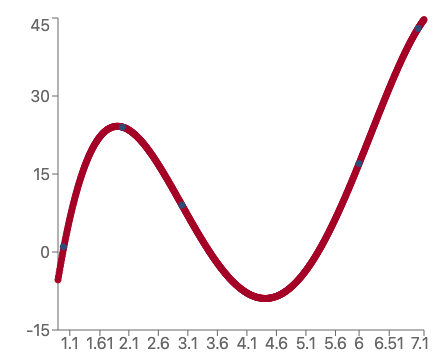# Quartic Regression Calculator

Created by Steven Wooding
Last updated: Aug 17, 2022

The quartic regression calculator applies a 4th-order polynomial model to a set of x-y data. A higher order polynomial is suitable when your data is more complicated than a cubic, or quadratic equation can handle.

• What is the quartic regression formula?
• How to use this quartic regression calculator.
• What is the difference between linear and polynomial regression?

## Quartic regression formula

The quartic regression formula is a 4th-order polynomial, similar to the standard quadratic equation, but the two additional terms: $x^3$ and $x^4$. Here is the general quartic formula:

$\small\! y = a_4 x^4 + a_3 x^3 + a_2 x^2 + a_1 x + a_0$

Different complex lines with two turning points can be generated by changing the values $a_0$ through to $a_4$, an example of which is shown below.Graph of x versus y of some data fitted with a quartic regression model.

To obtain the values of these coefficients, an algorithm minimizes the difference between each data point's y-value and the y-value given by the quartic formula.

How good a quartic model fits your data is determined by all the errors between the model and the data and is called R2: For a perfect fit, R2 is equal to 1.

For more on the math behind quartic regression, please check out the polynomial regression calculator.

## How to use the quartic regression calculator

To get a quartic regression model for your dataset, type in the x and y coordinates of each point into the calculator. You will need to enter at least 5 data points, and the calculator can accept a maximum of 30 data points (x, y pairs).

The results section gives you all the coefficients of the quartic equation and how well the model fits the data.

You can use the advanced mode of the calculator to change the precision of the coefficients.

## Other regression calculators

Here are some other polynomial-based regression calculators that you might find useful:

## FAQ

### What is a quartic function?

A quartic function is a 4th-order polynomial, consisting of five terms:

y = a₄x⁴ + a₃x³ + a₂x² + a₁x + a₀

Such a function has three critical, or turning, points and four real roots (found at points where the function crosses the x-axis).

### What is the difference between linear and polynomial regression?

In linear regression, we assume that the data best fit a straight line. Polynomial regression uses higher order x-axis terms so that we can model more complex data.

Generally, if you get a poor fit between data and model, try the next order of polynomial regression or another function (for example, the exponential function).

Steven Wooding
Quartic regression model
y = a4x4 + a3x3 + a2x2 + a1x + a0
Enter your data (up to 30 points)
x₁
y₁
x₂
y₂
Enter at least 5 points (both x and y coordinates) to get your model.
People also viewed…

### Black Friday

How to get best deals on Black Friday? The struggle is real, let us help you with this Black Friday calculator!

### Body fat

Use the body fat calculator to estimate what percentage of your body weight comprises of body fat.

### Decimal odds

Use our decimal odds calculator to quickly find your total return in case of a winning bet.

### Point estimate

Point estimate calculator computes the "best guess" of an unknown population parameter.